GFG App
Open AppBrowser
Continue

# Primer CSS Layout width and height

Primer CSS is a free open-source CSS framework that is built with the GitHub design system to provide support to the broad spectrum of Github websites. It creates the foundation of the basic style elements such as spacing, typography, and color. This systematic method makes sure our patterns are steady and interoperable with every other. Its approach to CSS is influenced by Object-Oriented CSS principles, functional CSS, and BEM architecture. It is a highly reusable model.

Primer CSS framework provides layouts that can be changed using utilities like display, visibility, position, alignment, float, overflow, width, and height. In this article, we will learn to implement various width and height utilities for layout documents.

Primer CSS Layout width and height classes:

• width-fit: This class is used to set max-width 100%.
• width-full: This class is used to set width to 100%.
• width-auto: This class is used to reset width to the initial value automatically. This is generally used for the responsive feature.
• height-fit: This class is used to set max height 100%.
• height-full: This class is used to set the height to 100%.

Syntax:

`<div class="Layout width and height class"> ...</div>`

Example 1: The following example demonstrates the width-fit class to set max-width 100% for an image resource.

## HTML

 ` ` `<``html` `lang``=``"en"``> ` `<``head``> ` `    ``<``meta` `charset``=``"UTF-8"` `/> ` `    ``<``meta` `http-equiv``=``"X-UA-Compatible"` `content``=``"IE=edge"` `/> ` `    ``<``meta` `name``=``"viewport"` `content``= ` `        ``"width=device-width, initial-scale=1.0"` `/> ` `     `  `    ``<``link` `rel``=``"stylesheet"` `href``= ` `"https://unpkg.com/@primer/css@^18.0.0/dist/primer.css"` `/>   ` `    ``<``style``> ` `      ``body ` `      ``{ ` `        ``margin-left:20px; ` `        ``margin-right:20px; ` `      ``} ` `    ``     ` ` ` ` `  `<``body``> ` `    ``<``div` `class``=``"o-container"` `style``=``"padding:1em;"``> ` `        ``<``h1` `class``=``"color-fg-success"``>  ` `            ``GeeksforGeeks  ` `        ``   ` `        ``<``h4` `class``=``"font-bold"``> ` `            ``Primer CSS Layout full width ` `        `` ` `        ``<``strong``>Set Full width of image to 100% ` `        ``<``div` `class``=``"one-fourth column"``> ` `              ``<``img` `class``=``"width-fit color-bg-subtle"` `src``= ` ` ``"https://media.geeksforgeeks.org/wp-content/uploads/20220522122914/geeksimage-200x145.png"`  `               ``alt``=``"geeksLogo"` `/> ` `        ``         ` `    `` ` ` ` ` `

Output: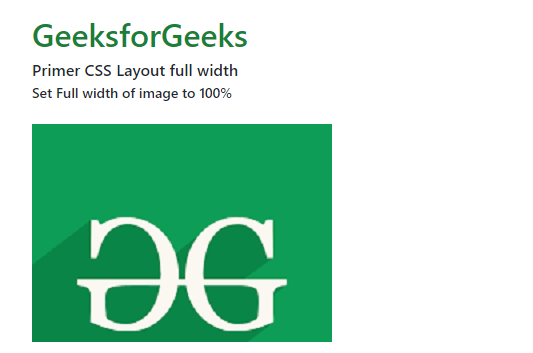Example 2: The following example demonstrates the width-fit class to set the width to 100% for a form field.

## HTML

 ` ` `<``html` `lang``=``"en"``> ` `<``head``> ` `    ``<``meta` `charset``=``"UTF-8"` `/> ` `    ``<``meta` `http-equiv``=``"X-UA-Compatible"` `content``=``"IE=edge"` `/> ` `    ``<``meta` `name``=``"viewport"` `          ``content``=``"width=device-width, initial-scale=1.0"` `/> ` `     `  `    ``<``link` `rel``=``"stylesheet"`  `          ``href``= ` `"https://unpkg.com/@primer/css@^18.0.0/dist/primer.css"` `/>   ` `    ``<``style``> ` `      ``body ` `      ``{ ` `        ``margin-left:20px; ` `        ``margin-right:20px; ` `      ``} ` `    ``     ` ` ` ` `  `<``body``> ` `    ``<``div` `class``=``"o-container"` `style``=``"padding:1em;"``> ` `        ``<``h1` `class``=``"color-fg-success"``>  ` `            ``GeeksforGeeks  ` `        ``   ` `        ``<``h4` `class``=``"font-bold"``> ` `            ``Primer CSS Layout full width ` `        `` ` `        ``<``strong``>Set Full width of form field to 100% ` `        `` ` `        ``<``div` `class``=``"d-table width-full"``> ` `            ``<``div` `class``=``"d-table-cell"``> ` `                ``<``input` `class``=``"form-control width-full"` `type``=``"text"`  `                       ``value``=``"Full width form field"`  `                       ``aria-label``=``"Sample full width form field"``> ` `            `` ` `        ``     ` `    `` ` ` ` ` `

Output: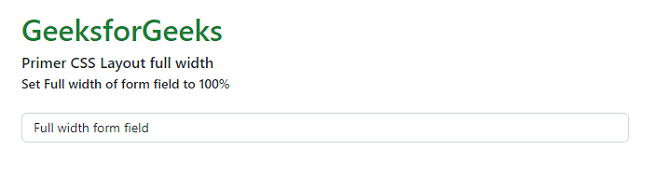Example 3: The following example demonstrates the width-full and width-md-auto class to set a responsive form field.

## HTML

 ` ` `<``html` `lang``=``"en"``> ` `<``head``> ` `    ``<``meta` `charset``=``"UTF-8"` `/> ` `    ``<``meta` `http-equiv``=``"X-UA-Compatible"` `content``=``"IE=edge"` `/> ` `    ``<``meta` `name``=``"viewport"` `          ``content``=``"width=device-width, initial-scale=1.0"` `/> ` `    ``<``link` `rel``=``"stylesheet"` `          ``href``= ` `"https://unpkg.com/@primer/css@^18.0.0/dist/primer.css"` `/>   ` `    ``<``style``> ` `      ``body ` `      ``{ ` `        ``margin-left:20px; ` `        ``margin-right:20px; ` `      ``} ` `    ``     ` ` ` ` `  `<``body``> ` `    ``<``div` `class``=``"o-container"` `style``=``"padding:1em;"``> ` `        ``<``h1` `class``=``"color-fg-success"``>  ` `            ``GeeksforGeeks  ` `        ``   ` `        ``<``h4` `class``=``"font-bold"``> ` `            ``Primer CSS Layout full width ` `        `` ` `        ``<``strong``>Set Responsive width of form field ` `        `` ` `        ``<``div` `class``=``"d-table width-full width-md-auto"``> ` `          ``<``div` `class``=``"d-table-cell"``> ` `            ``<``input` `class``=``"form-control width-full"` `type``=``"text"`  `                   ``value``=``"Responsive width form field"`  `                   ``aria-label``=``"Sample responsive width form field"``> ` `          `` ` `        `` ` `    `` ` ` ` ` `

Output: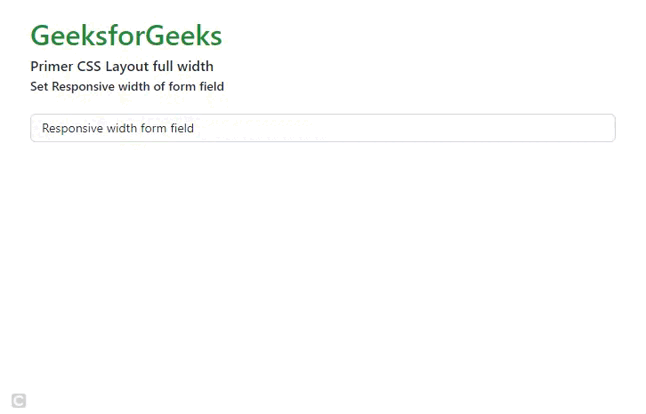Example 4: The following example demonstrates the height-fit class to set max-height 100% for an HTML div.

## HTML

 ` ` `<``html` `lang``=``"en"``> ` `<``head``> ` `    ``<``meta` `charset``=``"UTF-8"` `/> ` `    ``<``meta` `http-equiv``=``"X-UA-Compatible"` `content``=``"IE=edge"` `/> ` `    ``<``meta` `name``=``"viewport"`  `          ``content``=``"width=device-width, initial-scale=1.0"` `/> ` `    ``<``link` `rel``=``"stylesheet"` `          ``href``= ` `"https://unpkg.com/@primer/css@^18.0.0/dist/primer.css"` `/>   ` `    ``<``style``> ` `      ``body ` `      ``{ ` `        ``margin-left:20px; ` `        ``margin-right:20px; ` `      ``} ` `    ``     ` ` ` ` `  `<``body``> ` `    ``<``div` `class``=``"o-container"` `style``=``"padding:1em;"``> ` `        ``<``h1` `class``=``"color-fg-success"``>  ` `            ``GeeksforGeeks  ` `        ``   ` `        ``<``h4` `class``=``"font-bold"``> ` `            ``Primer CSS Layout full height ` `        `` ` `        ``<``strong``>Set full height to max-height 100% ` `        `` ` `        ``<``div` `class``=``"one-fourth column"`  `             ``style``=``"height:100px;overflow:auto;"``> ` `            ``<``div` `class``=``"p-3 height-fit border"``> ` `                ``Data structures are an integral part of Computers ` `                ``used for the arrangement of data in memory. ` `                ``They are essential and responsible for organizing, ` `                ``processing, accessing, and storing data efficiently.  ` `                ``But this is not all. Various types of Data Structures  ` `                ``have their characteristics, features, applications, ` `                ``advantages, and disadvantages. So how do you choose  ` `                ``which data structure to choose for a particular task? ` `                ``What is meant by the term Data structure? How many types ` `                ``of data structures are there and what are they used for? ` `            `` ` `        `` ` `    `` ` ` ` ` `

Output: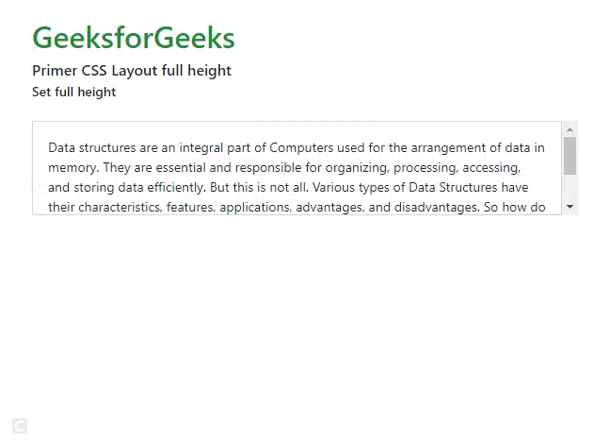Example 5: The following example demonstrates the height-full class to set the height to 100% for an HTML div.

## HTML

 ` ` `<``html` `lang``=``"en"``> ` `<``head``> ` `    ``<``meta` `charset``=``"UTF-8"` `/> ` `    ``<``meta` `http-equiv``=``"X-UA-Compatible"` `content``=``"IE=edge"` `/> ` `    ``<``meta` `name``=``"viewport"`  `          ``content``=``"width=device-width, initial-scale=1.0"` `/> ` `    ``<``link` `rel``=``"stylesheet"` `          ``href``= ` `"https://unpkg.com/@primer/css@^18.0.0/dist/primer.css"` `/>  ` `    ``<``style``> ` `      ``body ` `      ``{ ` `        ``margin-left:20px; ` `        ``margin-right:20px; ` `      ``} ` `    ``     ` ` ` ` `  `<``body``> ` `    ``<``div` `class``=``"o-container"` `style``=``"padding:1em;"``> ` `        ``<``h1` `class``=``"color-fg-success"``>  ` `            ``GeeksforGeeks  ` `        ``   ` `        ``<``h4` `class``=``"font-bold"``> ` `            ``Primer CSS Layout full height ` `        `` ` `        ``<``strong``>Set height to 100% ` `        `` ` `        ``<``div` `class``=``"d-table border"``> ` `            ``<``div` `class``=``"d-table-cell height-full v-align-middle pl-3"``> ` `                ``` `                ``<``svg` `class``=``"octicon octicon-three-bars"` `viewBox``=``"0 0 12 16"`  `                     ``version``=``"1.1"` `width``=``"12"` `height``=``"16"` `aria-hidden``=``"true"``> ` `                     ``<``path` `fill-rule``=``"evenodd"` `d= ` `                     ``"M11.41 9H.59C0 9 0 8.59 0 8c0-.59 0-1  ` `                     ``.59-1H11.4c.59 0 .59.41.59 1 0 .59 0 1-.59  ` `                     ``1h.01zm0-4H.59C0 5 0 4.59 0 4c0-.59 0-1  ` `                     ``.59-1H11.4c.59 0 .59.41.59 1 0 .59 0 1-.59  ` `                     ``1h.01zM.59 11H11.4c.59 0 .59.41.59 1 0 .59  ` `                     ``0 1-.59 1H.59C0 13 0 12.59 0 12c0-.59 0-1 .59-1z"> ` `                     `` ` `                `` ` `            `` ` `            ``<``div` `class``=``"p-3"``> ` `                ``The term PHP is an acronym for Hypertext Preprocessor.  ` `                ``It is a server-side scripting language that  ` `                ``is used for web development.  ` `                ``It can be easily embedded with HTML files. ` `                ``HTML codes can also be written in a PHP file.                  ` `                ``The PHP codes are executed on the server-side  ` `                ``whereas HTML codes are directly executed on the browser.                 ` `            `` ` `        `` ` `    `` ` ` ` ` `

Output: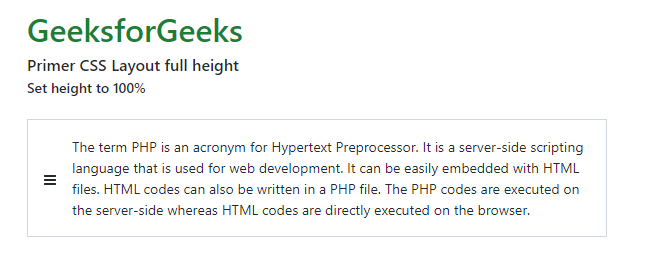My Personal Notes arrow_drop_up